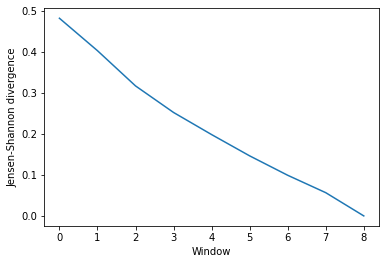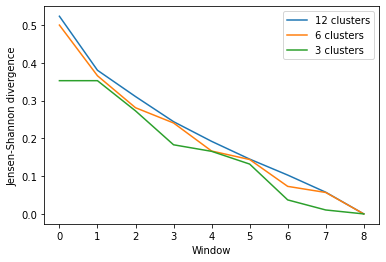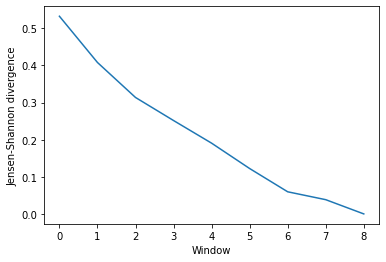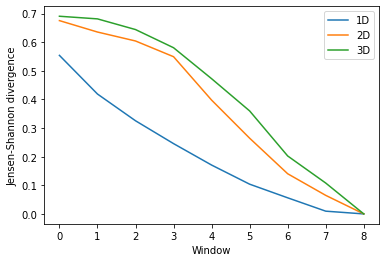# Evaluating convergence¶

Here we evaluate the convergence of a trajectory using the clustering ensemble similarity method and the dimensionality reduction ensemble similarity methods.

Last executed: Feb 06, 2020 with MDAnalysis 0.20.2-dev0

Last updated: January 2020

Minimum version of MDAnalysis: 0.20.1

Packages required:

Optional packages for visualisation:

Note

The metrics and methods in the encore module are from ([TPB+15]). Please cite them when using the MDAnalysis.analysis.encore module in published work.

:

import MDAnalysis as mda
from MDAnalysis.tests.datafiles import PSF, DCD
from MDAnalysis.analysis import encore
from MDAnalysis.analysis.encore.clustering import ClusteringMethod as clm
from MDAnalysis.analysis.encore.dimensionality_reduction import DimensionalityReductionMethod as drm

import numpy as np
import matplotlib.pyplot as plt
%matplotlib inline


The test files we will be working with here feature adenylate kinase (AdK), a phosophotransferase enzyme. ([BDPW09])

:

u = mda.Universe(PSF, DCD)


## Evaluating convergence with similarity measures¶

The convergence of the trajectory is evaluated by the similarity of the conformation ensembles in windows of the trajectory. The trajectory is divided into windows that increase by window_size frames. For example, if your trajectory had 13 frames and you specified a window_size=3, your windows would be:

- Window 1: ---
- Window 2: ------
- Window 3: ---------
- Window 4: -------------


Where - represents 1 frame.

These are compared using either the similarity of their clusters (ces_convergence) or their reduced dimension coordinates (dres_convergence). The rate at which the similarity values drop to 0 is indicative of how much the trajectory keeps on resampling the same regions of the conformational space, and therefore is the rate of convergence.

### Using default arguments with clustering ensemble similarity¶

See clustering_ensemble_similarity.ipynb for an introduction to comparing trajectories via clustering.

:

ces_conv = encore.ces_convergence(u,  # universe
10,  # window size
selection='name CA')  # default


The output is an array of similarity values, with the shape (number_of_windows, number_of_clustering_methods).

:

ces_conv

:

array([[0.48194205],
[0.40284672],
[0.31699026],
[0.25220447],
[0.19829817],
[0.14642725],
[0.09911411],
[0.05667391],
[0.        ]])


This can be easily plotted as a line.

:

ces_fig, ces_ax = plt.subplots()
plt.plot(ces_conv)
ces_ax.set_xlabel('Window')
ces_ax.set_ylabel('Jensen-Shannon divergence')

:

Text(0, 0.5, 'Jensen-Shannon divergence')### Comparing different clustering methods¶

You may want to try different clustering methods, or use different parameters within the methods. encore.ces_convergence allows you to pass a list of clustering_methods to be applied, much like normal clustering ensemble similarity methods.

Note

To use the other ENCORE methods available, you need to install scikit-learn.

The KMeans clustering algorithm separates samples into $$n$$ groups of equal variance, with centroids that minimise the inertia. You must choose how many clusters to partition. (See the scikit-learn user guide for more information.)

:

km1 = clm.KMeans(12,  # no. clusters
init = 'k-means++',  # default
algorithm="auto")    # default

km2 = clm.KMeans(6,  # no. clusters
init = 'k-means++',  # default
algorithm="auto")    # default

km3 = clm.KMeans(3,  # no. clusters
init = 'k-means++',  # default
algorithm="auto")    # default


When we pass a list of clustering methods to encore.ces_convergence, the similarity values get saved in ces_conv2 in order.

:

ces_conv2 = encore.ces_convergence(u,  # universe
10,  # window size
selection='name CA',
clustering_method=[km1, km2, km3]
)
ces_conv2.shape

:

(9, 3)


As you can see, the number of clusters partitioned by KMeans has an effect on the resulting rate of convergence.

:

labels = ['12 clusters', '6 clusters', '3 clusters']

ces_fig2, ces_ax2 = plt.subplots()
for data, label in zip(ces_conv2.T, labels):
plt.plot(data, label=label)
ces_ax2.set_xlabel('Window')
ces_ax2.set_ylabel('Jensen-Shannon divergence')
plt.legend()

:

<matplotlib.legend.Legend at 0x11f47df28>### Using default arguments with dimension reduction ensemble similarity¶

See dimension_reduction_ensemble_similarity.ipynb for an introduction on comparing trajectories via dimensionality reduction.

:

dres_conv = encore.dres_convergence(u,  # universe
10,  # window size
selection='name CA')  # default


Much like ces_convergence, the output is an array of similarity values.

:

dres_conv

:

array([[0.53383413],
[0.41463847],
[0.30425993],
[0.24385849],
[0.18008969],
[0.12072474],
[0.05691098],
[0.02318104],
[0.        ]])

:

dres_fig, dres_ax = plt.subplots()
plt.plot(dres_conv)
dres_ax.set_xlabel('Window')
dres_ax.set_ylabel('Jensen-Shannon divergence')

:

Text(0, 0.5, 'Jensen-Shannon divergence')### Comparing different dimensionality reduction methods¶

Again, you may want to compare the performance of different methods.

Principal component analysis uses singular value decomposition to project data onto a lower dimensional space. (See the scikit-learn user guide for more information.)

The method provided by MDAnalysis.encore accepts any of the keyword arguments of sklearn.decomposition.PCA except n_components. Instead, use dimension to specify how many components to keep.

:

pc1 = drm.PrincipalComponentAnalysis(dimension=1,
svd_solver='auto')
pc2 = drm.PrincipalComponentAnalysis(dimension=2,
svd_solver='auto')
pc3 = drm.PrincipalComponentAnalysis(dimension=3,
svd_solver='auto')

:

dres_conv2 = encore.dres_convergence(u,  # universe
10,  # window size
selection='name CA',
dimensionality_reduction_method=[pc1, pc2, pc3]
)
dres_conv2.shape

:

(9, 3)


Again, the size of the subspace you choose to include in your similarity comparison, affects the apparent rate of convergence over the trajectory.

:

labels = ['1D', '2D', '3D']

dres_fig2, dres_ax2 = plt.subplots()
for data, label in zip(dres_conv2.T, labels):
plt.plot(data, label=label)
dres_ax2.set_xlabel('Window')
dres_ax2.set_ylabel('Jensen-Shannon divergence')
plt.legend()

:

<matplotlib.legend.Legend at 0x1257dcb38>